# 17 – Matrices Redux

 < ρℝεν | ℂσητεητs | ℕεχτ >“All matter originates and exists only by virtue of a force… We must assume behind this force the existence of a conscious and intelligent Mind. This Mind is the matrix of all matter.” – Max Planck

After Chapter 15 and Chapter 16 we hopefully have a reasonable sense of Vector Spaces. Earlier, in Chapter 5 and Chapter 6, we introduced matrices and the concept has permeated much of what we have looked at since .

Before plunging into the next part of the book, which begins with Chapter 18 and relates to Lie Algebras and Lie Groups, I am going to pause to develop some more apparatus pertaining to matrices. These are properties which we will reuse later as we begin to talk a bit more about both Lie Groups and Lie Algebras and their pertinence to Particle Physics. These concepts may at first seem of limited relevance, but – as well as being fundamental in Mathematics – they provide one link between how matrices represent certain aspects of particles and physically measurable quantities; something we touch on here and develop further later.

However, I’m going to start by looking at something that – at first sight – probably appears a world away from Particle Physics, some specific combinations of matrices and vectors and what happens when we multiply them.

More Marvellous Matrix MultiplicationsIn passing we have mentioned that matrices may act on vectors, by which we mean multiplication. Rather than defining something new, if we simply think of a vector as being represented by an n × 1 matrix, where n is the dimension of the Vector Space, then our vector would look like:and we are simply in the familiar realm of matrix multiplication. We could pre-multiply an n × 1 matrix by any m × n matrix or post-multiply it by any 1 × p matrix . Here we are going to specifically focus on pre-multiplying by square n × n matrices.

If we start, as we generally do, with the simplest case n = 2 , then we will be talking about multiplying vectors with two elements by 2 × 2 matrices. Rather than worrying about abstract Vector Spaces, let’s just consider 2D Euclidean space with an x- and a y-axis. Then all our numbers are Real and the operation we are considering is:Which we can write as:

Mv = v′

Where M is the 2 × 2 matrix with entries {a, b, c, d}, v is the vector (vx, vy) and v′ is the result of the multiplication, ie. v′ = (avx + bvy, cvx + dvy)

Let us consider what happens to a specific vector when multiplied by a specific matrix. For example if M is the Identity Matrix, I, then v = v′, for all v. Equally if M is 2 times the Identity Matrix, then 2v = v′, for all v.

However, more generally, depending on the attributes of the matrix, M, and the attributes of the vector, v, the resulting vector v′ might have a whole range of properties. Importantly, some vectors might be radically altered by a matrix, whereas others may remain constant, or change in a more limited manner.

If we consider a matrix, which carries out a reflection in the y-axis when pre-multiplied, then we may recall from Chapter 6 that this looks like:If we consider a specific vector, (0, 1), we can see that:I.e. multiplication by our reflection matrix results in no change to this vector. A moment′s thought will lead us to the conclusion that the same holds for any vector (0, vy). Such vectors are identical to (0, 1) save for multiplication by some scalar.

Let′s instead consider a different matrix as follows: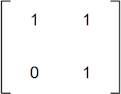Rather than looking at its action on a single vector, let’s consider three vectors, (1, 0), (1, 1) and (0, 1), which, together with the origin, form the vertices of a square as below:Our latest matrix acts on these vectors as follows:Which means our diagram is transformed to become: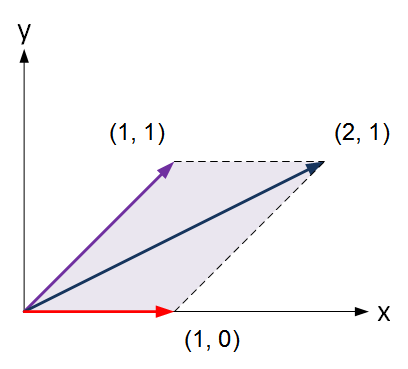This has obviously skewed the square to the right. However, it may be noted that while vectors (1, 1) and (0, 1) have both been changed, the vector (1, 0) has been undisturbed by the transformation.

Let’s consider one further extension of this before moving on to some general definitions. This is the matrix: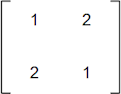Again let’s see what this does to the same square we started with beforehand:This transforms our starting square into the following shape: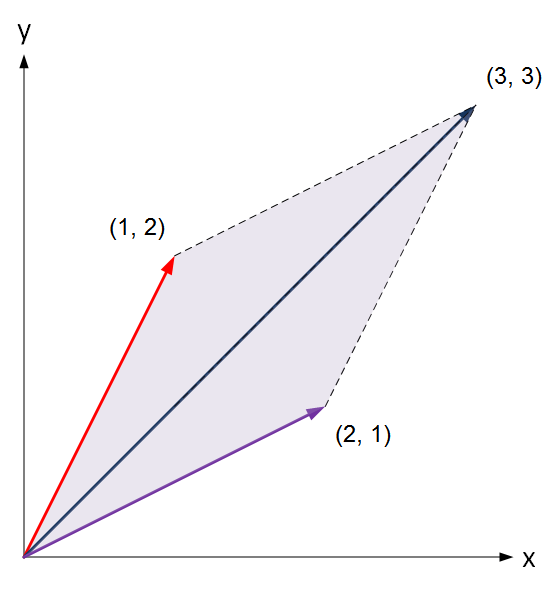Here we have a skew in both the vertical and horizontal directions, the square has grown and (noting that the red vector is now at the top and the purple one at the bottom) also flipped over. This is quite a dramatic change and none of our original vectors have been left unscathed. However we may note that (1, 1) has been stretched into (3, 3) but it is only its magnitude that has changed, not its direction.

It seems in each of our examples that we could find a vector that was either unchanged by the specific matrix multiplication, or that was only changed by a scalar factor. For a given matrix, such special vectors are know as Eigenvectors and the scalar factors are know as Eigenvalues. The German word eigen means “own”, so these terms can be thought of as a matrix’s own vectors and values, the ones particular to this matrix. Having given at least a flavour of what these concepts mean, let’s proceed to define them more rigorously and to extend the concept to n × n matrices.

Establishing OwnershipHere I’m going to elide details about Vector Spaces and the like and focus on the essentials.

For an n × n matrix, M, a non-zero vector, v, and a possibly zero scalar, λ, are said to be an eigenvector and eigenvalue of M respectively if:

Mv = λv

That is if multiplying the vector by the matrix has the same effect as multiplying it by a scalar, leaving its main characteristic (its direction) unchanged; or possibly reversed if the scalar is negative.

We can rearrange the above to note that this is the same as requiring that:

Mv – λv = 0

or

(M – λI)v = 0

Where I is the n × n identity matrix.

In the definition, we said that it was a requirement that v cannot be zero, so let’s focus on (M – λI). To save us some complexities, let’s call this matrix A, so:

Av = 0

What happens if we assume that A is invertible? Then there exists a non-zero A-1 such that AA-1 = A-1A = I. If we multiply both sides of our equation by A-1, we get:

A-1Av = A-10 = 0

As anything times the zero matrix is the zero matrix. Then:

Iv = v = 0

We therefore conclude that A cannot be invertible, which is precisely equivalent to stating that the determinant of A must be zero, so we can write our eigenvector / value requirement as:

det(M – λI) = 0

To date I have rather steadfastly refused to give a generic definition of a determinant, one that holds for matrices bigger than n × n . I’m not going to change my approach here and will instead state that, for an n × n matrix, M, det(M – λI) = 0 may be rewritten as a polynomial of degree n in λ. That is:

anλn + an-1λn-1 + … + a1λ + a0 = 0

Such an equation is known as the Characteristic Polynomial of the matrix.

From our work with polynomials in Chapter 11, we know that a polynomial of degree n has precisely n roots (some of which may of course be repeats of each other). If we label these roots λ1, λ2, … , λn, then we can rewrite our Characteristic Polynomial as:

(λ – λ1)(λ – λ2) … (λ – λn) = 0

The λi are the eigenvalues of M and we have just shown that an n × n matrix has n of them (again possibly with some repeats). Given that we are dealing with a polynomial, Chapter 11 will also bring to mind that – even if all of our matrix entries are Real and thus all coefficients in our polynomial are also real – some of our eigenvalues may be complex.

 Note: A Good Characteristic A famous result in Linear Algebra is the Cayley-Hamilton Theorem, which states that any n × n matrix, M, satisfies its own Characteristic Polynomial. So, if we take the example from above where the eigenvalues of M are λ1, λ2, … , λn and thus the Characteristic Polynomial of M is: (λ – λ1)(λ – λ2) … (λ – λn) = 0 We also have: (M – λ1I)(M – λ2I) … (M – λnI) = 0 Where I is the n × n identity matrix. Hamilton published results which related to special cases of 2 × 2 and 4 × 4 matrices in 1853. Cayley demonstrated that the equation held for 2 × 2 and 3 × 3 matrices in 1858. However it was Frobenius who proved the general theorem in 1878. For the same reasons that Fermat’s Last Theorem will never be known as Wiles’s Theorem, the name Cayley-Hamilton stuck.

Let’s work through the process of determining eigenvalues and eigenvectors for the matrix we last used starting with the determinant of M – λI being zero: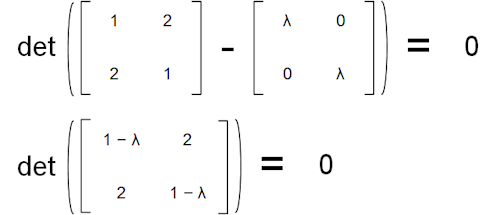Recalling that the determinant of a 2 × 2 matrix  with entries {a, b, c, d} is simply equal to ad – bc, we have:

(1 – λ)(1 – λ) – 4 = 0

Which we can rearrange as:

λ2 – 2λ – 3 = 0

Which factors as:

(λ + 1)(λ – 3) = 0

Which of course has two roots, λ = – 1 and λ = 3.

These two roots are our eigenvalues. What about the eigenvectors? Let’s plug our values for λ back into the equation: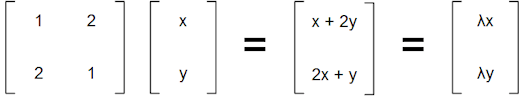When λ = -1 we have:

x + 2y = – x

2x + y = – y

Both of which simply tell us that x = – y.

So as long as our two vector entries preserve this property, it doesn’t matter if we pick (1, -1), (10, – 10) or ( 3√(πe – 5)/422, – 3√(πe – 5)/422 ), they will all work, being scalar multiples of each other. If the direction of a vector is not changed by matrix multiplication, then the direction of any scalar multiple of it will also not be changed.

In general there is not a unique eigenvector for an eigenvalue, but instead a unique direction. We tend to pick a vector that makes sense, here (1, – 1) is the obvious choice.

When λ = 3 we have:

x + 2y = 3x

2x + y = 3y

Both of which simply tell us that x = y.

So we can pick as our second eigenvector (1, 1), which is the blue vector we saw was broadly unchanged in our diagram above.

Let’s just double check that our logic has been sound, by multiplying each of these eigenvectors by our matrix as follows:So we did our sums right, which is always comforting!

 Note: In a box above we introduced the Cayley-Hamilton Theorem, which states that any n × n matrix, M, satisfies its own Characteristic Polynomial. Let’s check this using our example. Here our Characteristic Polynomial is: (λ + 1)(λ – 3) = 0 So we need to consider the matrix equation: (M + 1I)(M – 3I) Where I is the 2 × 2 identity matrix. Using the actual matrices we have:Carrying out the additions and then the multiplication we get:Which is clearly the zero matrix, so our result holds.

Perhaps labouring a point, I mentioned above that if v is an eigenvector then so is λv where λ is a scalar. Let’s just demonstrate this explicitly by considering multiples of our two eigenvectors (1, – 1) and (1, 1). Let’s multiply the former by – 8 and the latter by 13; two numbers selected entirely at random. This gives us two other vectors, (- 8, 8) and (13, 13), then we have:Which demonstrates the point nicely. Everything that we have noted above about eigenvectors and eigenvalues for 2 × 2 matrices, scales up  to n × n ones as well.

Eigenlob für Eigenvalues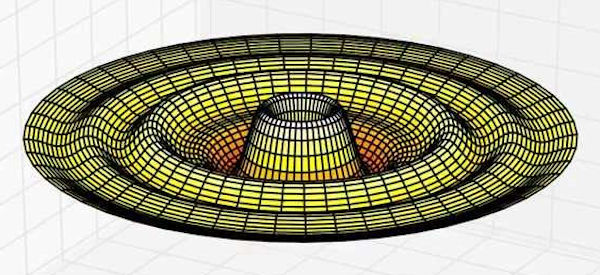Before closing this Chapter, it is worth catalouging some further properties of eigenvectors and eigenvalues; a couple of which we will rely upon in later work. I will prove some of the results, I will state some without proof and for some I will just provide a broad indication of a proof. Here I have adopted the standard Indiana Jones / Harry Potter nomenclature.

Eigenvalues and the Inverse of a matrix

If we take the canonical definition of eigenvectors and eigenvalues for a matrix, M, and further assume that M is invertible, so there exists, M-1 such that MM-1 = M-1M = I, then we can see that:

Mv = λv

Multiply both sides by M-1:

M-1Mv = λM-1v

So:

v = λM-1v

Which we can rearrange as:

(1/λ)v = M-1v

Which implies that v is also an eigenvector of M-1 with eigenvalue 1/λ.

In general, if the eigenvalues of an n × n invertible matrix, M, are λ1, λ2, …, λn, then the eigenvalues of M-1 are 1/λ1, 1/λ2, …, 1/λn.

Eigenvalues and the Transpose of a matrix

If we use our previous notation of MT for the transpose of a matrix, M , then:

It can be shown that det(M) = det(MT)  and it is also easy to see that (M + N)T = MT + NT.

Then, for a matrix, M, the Characteristic Polynomial of its transpose, MT, is given by:

det(MT – λI) = 0

We can easily see that (λI)T = λI, which holds for any purely diagonal matrix. Then, using the second property above, we have:

MT – λI = MT – (λI)T = (M – λI)T

so:

det(MT – λI) = det[(M – λI)T]

Using the first property:

det[(M – λI)T] = det(M – λI)

So M and MT have the same Characteristic Polynomial and therefore the same eigenvalues.

In general a matrix and its transpose have the same eigenvalues.

Eigenvalues and the Conjugate Transpose of a matrix

We will recall that MH is the notation used for the conjugate transpose of M , then:

Again it may be shown that that det(MH) = det(M)  and, as with transposes, it is also easy to see that (M + N)H = MH + NH.

Then, for a matrix, M, the Characteristic Polynomial of its, conjugate transpose, MH is given by:

det(MH – λI) = 0

Again it is straightforward to note that (λI)H = λ I and symmetrically that (λI)H = λI so:

MH – λI = MH – (λI)H

Which by our second property means that:

MH – (λI)H = (M – λI)H

So:

det(MH – λI) = det[(M – λI)H]

By our first property we can then note that:

det[(M – λI)H] = det(M – λI)

Which is clearly the Characteristic Polynomial of M, but with the eigenvalues transformed to their complex conjugates.

In general the eigenvalues of the conjugate transpose of a matrix are the conjugates of the eigenvalues of the original matrix.

Eigenvalues and the Determinant of a matrix

We used the determinant to help us find the n eigenvalues λ1, λ1, … , λn of an n × n matrix, M. This works the other way round as well, in particular:

det(M) = λ1λ1 … λn

So a matrix’s determinant is also the product of its eigenvalues.

As a matrix is invertible if an only if its determinant is non-zero, the above result implies that a matrix is invertible if and only if it has no zero eigenvalues.

Eigenvalues and the Trace of a matrix

In the next Chapter, we will meet another property of a square matrix, its trace. The trace of a matrix is the sum of the entries on its major diagonal, the one running from top left to bottom right. If we know the eigenvalues of a matrix, M, then we can calculate its trace as follows:

tr(M) = λ1 + λ1 + … + λn

So a matrix’s trace is also the sum of its eigenvalues.

 Note: Cayley-Hamilton by any other name The Cayley Hamilton Theorem states that any square matrix, M, satisfies its own Characteristic Polynomial, which is derived from the equation: det(M – λI) = 0 As ever, I shy away from explicit definitions of the determinant in this book, but, for the n × n case, the formula for the determinant of a matrix allows us to expand the above as a rather complex expression involving the trace of powers of M and the determinant of M. I have no intention of reproducing this here, but will show what it looks like for the cases n = 2 and n =3 below: n = 2 det(M2 – λI2) = λ2 – tr(M2)λ + det(M2) n = 3 det(M3 – λI3) = λ3 – tr(M3)λ2 + ½[tr(M3)2 – tr(M32)]λ – det(M3) The Cayley-Hamilton Theorem then tell us that if we substitute M3 for λ in the n = 3 result above, we get: M33 – tr(M3)M32 + ½[tr(M3)2 – tr(M32)]M3 – det(M3)I3 = 0 We will employ this specific result in Chapter 21.

Eigenvalues and the Hermitian matrices

Hermitian Matrices are ones whose conjugate transpose is the matrix itself, i.e. MH = M.

From a result above, if an eigenvalue of a Hermitian matrix, M, is λ, then the corresponding eigenvalue of its conjugate transpose, MH, is λ’s complex conjugate, λ. But MH = M and so, the eigenvalues of MH must be the same as those of M. The only way that a Complex Number and its complex conjugate can be equal is if the number is Real (i.e. it has no imaginary component).

So, while the eigenvalues of a Matrix may in general be Complex, eigenvalues of Hermitian matrices must all be Real. This is an important result because the eigenvalues of certain matrices used in Physics correspond to physical values such as energy (see the Note box below).

 Note: Eigenvectors and eigenvalues play a large role in Quantum Mechanics. Without getting into a primer on the subject , an important concept is the Hamiltonian of a quantum system. This captures the kinetic and potential energies of all particles in the system. The Hamiltonian is essentially a matrix  and its eigenvalues relate to specific measurable quantities of the system, such as the possible energy levels it can adopt. Given that Hamiltonians are Hermitian, the result we referenced above about the eigenvalues of a Hermitian matrix being Real comes into play here; ensuring we don’t get Complex energy. The following striking image and accompanying text is from Wikipedia.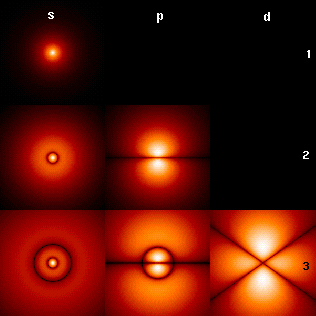The wavefunctions associated with the bound states of an electron in a hydrogen atom can be seen as the eigenvectors of the hydrogen atom Hamiltonian as well as of the angular momentum operator. They are associated with eigenvalues interpreted as their energies (increasing downward: 1, 2, 3) and angular momentum (increasing across: s, p, d). The illustration shows the square of the absolute value of the wavefunctions. Brighter areas correspond to higher probability density for a position measurement. The center of each figure is the atomic nucleus, a proton.

Eigenvalues and the Unitary matrices

Back in Chapter 13 we met Unitary matrices (which form Unitary Groups). The conjugate transpose of such a matrix, M, is its inverse. So MHM = I. What can we say about the eigenvalues of such matrices?

Well if λ is a eigenvalue of a Unitary matrix, M, then we showed above that corresponding eigenvalue of its conjugate transpose, MH, is λ’s complex conjugate, λ. But MH = M-1 and again above we showed that the corresponding eigenvalue of M-1 is 1/λ.

This means that:

λ = 1/λ

Which we can rearrange to give:

λ λ = 1

From Chapter 14 we can recall that for any complex number, z, we have:

z z = |z|2

So this means that λ must have an absolute size of 1.

In general this means that all eigenvalues of a Unitary matrix fall in the unit circle on the Complex Plane; which is of course all Complex numbers whose absolute size is equal to 1.

Given what we have trailed as the role of Unitary (and Special Unitary) Groups in Particle Physics. This result is also of non-Mathematical interest because it means that multiplying a vector by Unitary matrices preserves certain aspects of measurements of distance .

So we have spent some time defining eigenvectors and eigenvalues and exploring their sometimes surprising and often beautiful properties. As hinted in the text above, we will return to the subject of eigenvectors and eigenvalues later in this book. However, it is now time to return to our primary objective in studying Vector Spaces, to define and better understand Lie Algebras and, by this, Lie Groups. It is to this task that we turn our attention in the next four Chapters, which commence with Chapter 18.

 Concepts Introduced in this Chapter Eigenvectors / values of a matrix For a matrix, M, a non-zero vector, v, and a scalar, λ, such that: Mv = λv That is, at least to some extent, the eigenvector is invariant under the action of the matrix; at most having its magnitude increased or decreased by a scalar factor. Characteristic Polynomial For an n × n matrix, M, a polynomial formed by considering the determinant of a matrix derived from the definition of eigenvectors and values [i.e. (M – λI) where λ is any eigenvalue]. The n roots of this equation are the eigenvalues of M, which also demonstrates that an n × n matrix has precisely n eigenvectors / values. Cayley-Hamilton Theorem An n × n matrix satisfies its own Characteristic Polynomial. Trace as sum of eigenvalues For an n × n matrix, M, with eigenvalues λ1, λ1, … , λnwe have: trace(M) = λ1 + λ1 + … + λn Hermitian matrices Square Complex matrices that have the property that their conjugate transpose is equal to the matrix itself, so MH = M (see also Chapter 18). Eigenvalues of Hermitian matrices These are always Real Numbers, which is of pertinence in Quantum Mechanics and other parts of Physics. Eigenvalues of Unitary matrices These are always of size 1 and thus fall on the unit circle in the Complex Plain.
 < ρℝεν | ℂσητεητs | ℕεχτ >

Chapter 17 – Notes

  A hint, this is most likely going to continue to be the case going forwards, so probably worth getting used to the idea!  Where by “multiply” we mean standard matrix multiplication.  Recalling that n = 1 relates to numbers themselves, rather than tables of multiple numbers, and so is a trivial case.  Though such a definition does appear in the banner image at the start of Chapter 14 and is repeated below:Which I did deign to define back in Chapter 5.  No pun intended.  Eigenlob is self-praise in German. As in the adage Eigenlob stinkt, which may be translated idiomatically as “Don’t blow your own horn”, or literally as “Self-praise stinks”.  If the entries of our original matrix, M, are {aij}, where ≤ i, j ≤ n, then the entries in the transpose of M, MT, are {aji}. That is the matrix entries are swapped across the leading diagonal, which remains unchanged itself.  The formula for a determinant provided in Note 4 above provides a clue here. It can be seen that transposing the elements of a matrix simply changes the order of terms in the determinant formula without changing the overall sum. I.e. A + B + C + D = A + D + C + B.  First recall that the complex conjugate of a complex number a + ib is denoted by a bar and that then a + ib = a – ib. To create the conjugate transpose of a matrix, M, we take the complex conjugate of all entries of M, creating the conjugate matrix, M, and then form the transpose of M (or vice versa as the order in which the operations are performed is immaterial). So MH = MT. Again, if the original matrix, M, has entries {aij}, where 1 ≤ i, j ≤ n, then its conjugate transpose, MH, has entries {aji}.  As with Note 8 above, we can see that the complex conjugate of the overall expression for the determinant will be the complex conjugate of the individual terms because of helpful results like: ab = a b a + b = a + b and αb = αa (where α ∈ ℝ).  Please recall that this is a Mathematics book, not a Physics one.  It’s actually a Linear Operator, but the concepts are very closely related and Quantum Mechanics can be stated in matrix form. For Linear Operators, the analogue of an eigenvector is an eigenfunction (actually a specialised type of eigenvector), but eigenvalues retain the same name and both are defined and act in an identical manner as for matrices.  Using a more generalised and technical definition of distance that we won’t meet until Chapter 22.
 Text: © Peter James Thomas 2016-17. Images: © Peter James Thomas 2016-17, unless stated otherwise. Published under a Creative Commons Attribution 4.0 International License.